Question

A biased coin is tossed n times. The probability of heads is p and the probability of tails is q and p=2q. Choose all correct statements. This is an example of a Bernoulli trial n-n-1-1-(k-1) p...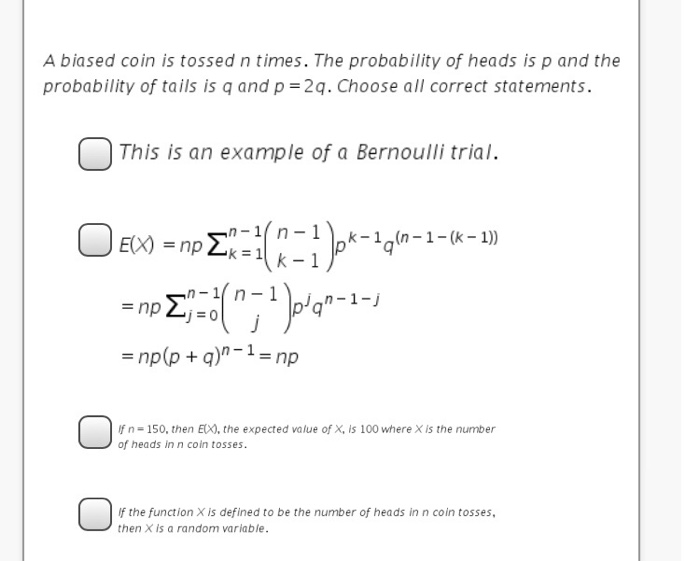A biased coin is tossed n times. The probability of heads is p and the probability of tails is q and p=2q. Choose all correct statements. This is an example of a Bernoulli trial n-n-1-1-(k-1) p'q =np(p + q)n-1 = np f n- 150, then EX), the expected value of X, is 100 where X is the number of heads in n coin tosses. f the function X is defined to be the number of heads in n coin tosses, then X is a random variable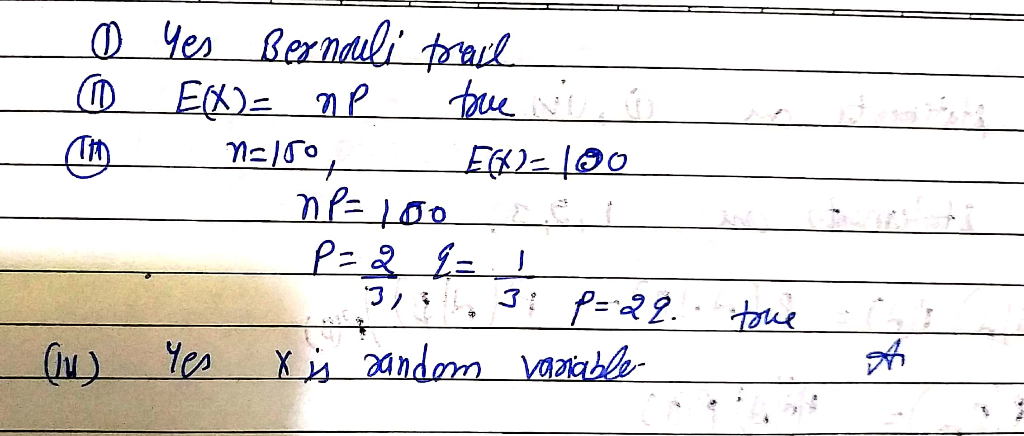Earn Coins

Coins can be redeemed for fabulous gifts.

Similar Homework Help Questions
• (Question Consider a biased coin where P(Head) = p = 1-q, i.e. it is Bern(p) This...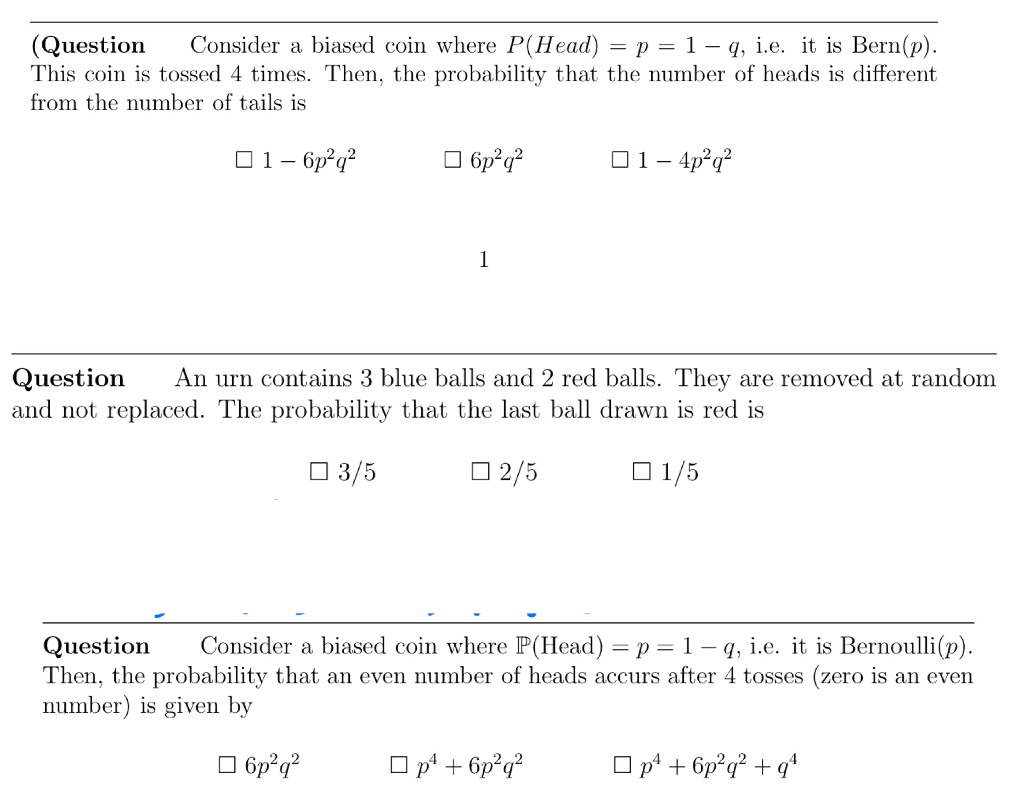(Question Consider a biased coin where P(Head) = p = 1-q, i.e. it is Bern(p) This coin is tossed 4 times. Then, the probability that the number of heads is different rom the number of tails is Question An urn contains 3 blue balls and 2 red balls. They are removed at random and not replaced. The probability that the last ball drawn is red is □ 3/5 □ 2/5 1/5 Question Consider a biased coin where P(Head)-p-1 -q, i.e....

• Consider a coin with probability q of landing on heads, and probability 1−q of landing on...

Consider a coin with probability q of landing on heads, and probability 1−q of landing on tails. a) The coin is tossed N times. What is the probability that the coin lands k times on heads. b) The coin is tossed 100 times, and lands on heads 70 times. What is the maximum likelihood estimate for q?

• A biased coin is tossed until a head occurs. If the probability of heads on any...

A biased coin is tossed until a head occurs. If the probability of heads on any given toss is .4, What is the probability that it will take 7 tosses until the first head occurs? The answer i got was , (.60)^2(.40) Now for the second part it says, what is the probability that it will take 9 tosses until the second head occurs. Is the answer for this part be 9C2(.40)^2(.60)^7 or 8C1(.40)(.60)^5 I can't figure out if its...

• 9) A fair coin is tossed n times, coming up Heads Nh times and Tails Nr...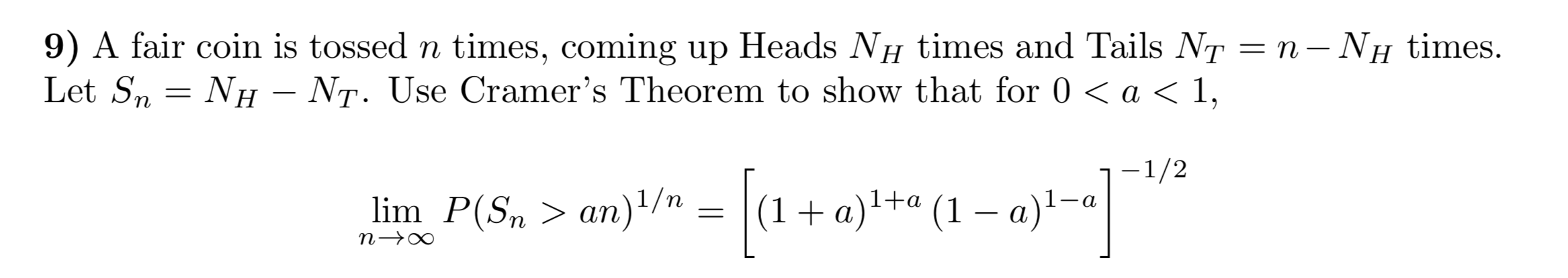9) A fair coin is tossed n times, coming up Heads Nh times and Tails Nr = n – Nh times. Let Sn = Nh – Nt. Use Cramer's Theorem to show that for 0 < a < 1, 1-1/2 lim n-> P(Sn. = ( + (1 - a)1-a

• A coin with probability p of heads is tossed until the first head occurs. It is...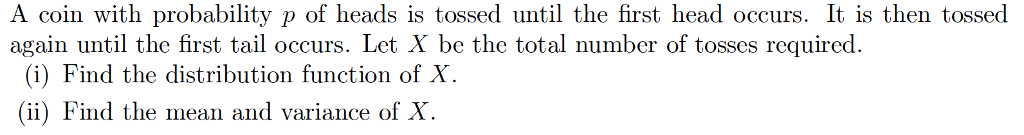A coin with probability p of heads is tossed until the first head occurs. It is then tossed again until the first tail occurs. Let X be the total number of tosses required. (i) Find the distribution function of X. (ii) Find the mean and variance of X

• A coin is tossed three times. X is the random variable for the number of heads...

A coin is tossed three times. X is the random variable for the number of heads occurring. a) Construct the probability distribution for the random variable X, the number of head occurring.             b) Find P(x=2).             c) Find P(x³1). d) Find the mean and the standard deviation of the probability distribution for the random                  variable X, the number of heads occurring.

• A coin with probability 0.8 of heads is tossed n times. Sn denotes the number of...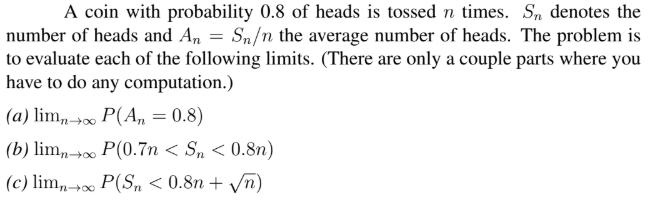A coin with probability 0.8 of heads is tossed n times. Sn denotes the number of heads and An - Sn/n the average number of heads. The problem is to evaluate each of the following limits. (There are only a couple parts where you have to do any computation.) (a) limP(A 08) (b) limn →00 P(0.7n < Sn < 0.8m) c) llmn00

• 4. You toss n coins, each showing heads with probability p, independently of the other tosses....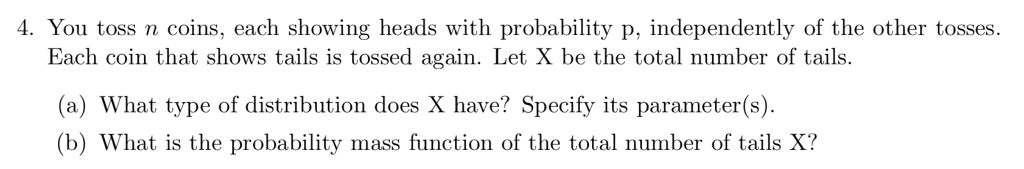4. You toss n coins, each showing heads with probability p, independently of the other tosses. Each coin that shows tails is tossed again. Let X be the total number of tails (a) What type of distribution does X have? Specify its parameter(s). (b) What is the probability mass function of the total number of tails X?

• Problem 4. A fair coin is tossed consecutively 3 times. Find the conditional probability P(A |...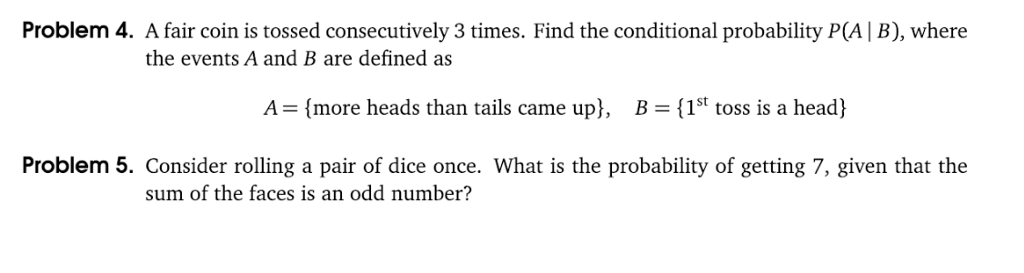Problem 4. A fair coin is tossed consecutively 3 times. Find the conditional probability P(A | B), where the events A and B are defined as A-(more heads than tails came upl, B-(1st toss is a head) 1St toss is a head Problem 5. Consider rolling a pair of dice once. What is the probability of getting 7, given that the sum of the faces is an odd number?

• Problem 7. Suppose that a coin will be tossed repeatedly 100 times; let N be the...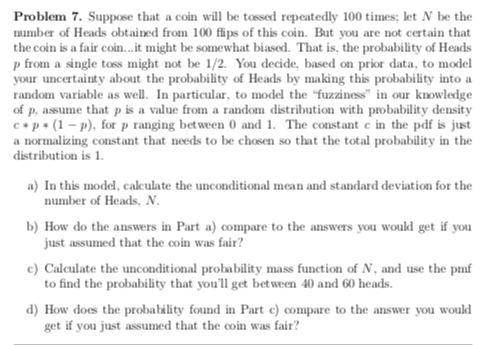Problem 7. Suppose that a coin will be tossed repeatedly 100 times; let N be the number of Heads obtained from 100 fips of this coin. But you are not certain that the coin is a fair coin.it might be somewhat biased. That is, the probability of Heads from a single toss might not be 1/2. You decide, based on prior data, to model your uncertainty about the probability of Heads by making this probability into random variable as wl....